## Understanding IBIS-AMI Simulations

IBIS-AMI models represent an alternative to the traditional approach to time-domain simulation. They can simulate a much larger number of bits and verify lower bit error rates. IBIS-AMI approach is based on two fundamental assumptions:

• You can break a transmitter or receiver into two independent parts:

• an analog part representing the output buffer and termination of the transmitter or an input termination of the receiver, and

• an algorithmic or digital part modeling the equalization capabilities of the device.

• The analog parts of the transmitter, receiver, and the passive interconnects are linear and time-invariant (LTI). This allows you to characterize them completely by a single impulse response. This path from output buffer through the passive interconnect to the input buffer is referred to as the analog channel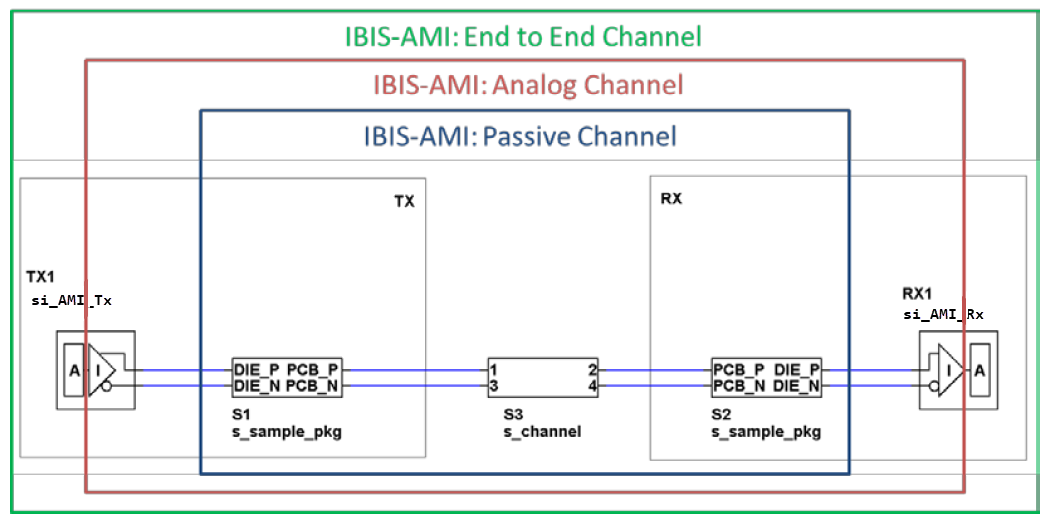Based on these two assumptions, you can create an eye diagram at the receiver in two different ways. Each method implies a different type of algorithmic model.

First, you can create a statistical eye at the receiver if the algorithmic portions of the transmitter (TX) and receiver (RX) are LTI and you can model them with an impulse response. The statistical simulation has no bit stream. Instead, it constructs an eye showing all combinations of bits capable of generating inter-symbol interference (ISI). The eye is constructed based solely on the impulse response of the channel and the principle of superposition. The eye represents the probability of the signal occurring throughout the unit interval (UI).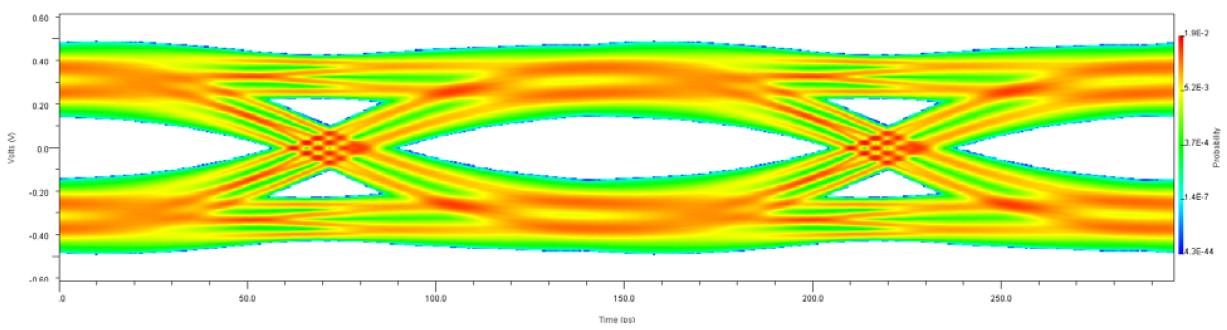You can also generate an eye diagram based on bit-by-bit time-domain simulation. Here, the models for algorithmic portions of the transmitter and receiver can be non-LTI. The models are provided with a bit stream by the electronic design automation (EDA) tool. The bit stream is modified according to the equalization capabilities of the model(s) before being returned to the EDA tool. The eye diagram is constructed by simply overlaying the individual bits. Since the time-domain simulation is carried out on an actual bit stream, you can also model other device features not related to equalization. For example, you can model the clock recovery and adaptive selection of the tap weights of a decision feedback equalizer.

Often, TX or RX models lack the necessary support for the bit-by-bit simulation. However, the EDA tool can use the impulse response of the model in a time-domain simulation through convolution of the impulse response with an input bit stream. This type of simulation is referred to as Init-based time-domain simulation. For more information, see Statistical Analysis in SerDes Systems.

### IBIS-AMI Models

According to the IBIS-AMI specification, SerDes executable transmitter or receiver models can contain up to three functions:

• `AMI_Init`, required function

• `AMI_GetWave`, optional function

• `AMI_Close`, required function

All equalization or clock recovery capabilities are modeled in the `AMI_Init` and `AMI_GetWave` functions. If you want to use the model for statistical simulations, `AMI_Init` accepts an input impulse response from the EDA tool, convolves it with the impulse response of the device, and returns the result. If you want to use the model for time-domain simulations, `AMI_GetWave` accepts an input bit stream, modifies it based on the equalization features of the device, and returns the modified bit stream to the EDA tool. You need an EDA tool to call the `AMI_Init` function of a model regardless of the type of simulation.

In order to convey the capabilities of a model to an EDA tool, the AMI specification defines two required boolean parameters:

• Init_Returns_Impulse — notifies the EDA tool whether the model supports the statistical flow.

• GetWave_Exists — indicates whether or not the AMI_GetWave function is available for time-domain simulations.

These parameters are included in the AMI file of the model. All AMI models can be classified as one of three types and the processing flow in a simulation is determined by the particular combination of TX and RX models.

Model TypeInit_Returns_ImpulseGetWave_ExistsSimulation Capabilities
Init-OnlyTrueFalseStatistical, Init-based time-domain
GetWave-OnlyFalseTrueGetWave-based time-domain
DualTrueTrueStatistical, GetWave-based time-domain

### Simulation Flow

The TX and RX models are completely independent. For example, if the TX model is of the Init-Only type, there is no requirement that the RX model also be Init-Only. The EDA tool must support all combinations of model types. There are nine possible combination of model types in a single link. The keywords used in simulation flow are:

KeywordDefinitionDescription
hAC(t)Impulse response of analog channel.Product of the network characterization phase of a simulation. Network characterization is required for both statistical and time-domain simulations.
hTE(t)Impulse response of TX equalization.LTI forms of TX equalizations.
hRE(t)Impulse response of RX equalization.LTI forms of RX equalizations.
gTE[t]GetWave-based TX equalization.Time-domain, possibly non-LTI, representations of TX equalizations.
gRE[t]GetWave-based RX equalization.Time-domain, possibly non-LTI, representations of RX equalizations.

#### Tx Init-Only and Rx Init-Only

Neither model has an `AMI_GetWave` function. So you can only perform statistical or Init-based time-domain simulations. The final eye diagrams from the two flows should be fairly similar since both are using the same, static equalization. Any difference is due to the finite number of bits in a time-domain simulation. A statistical eye shows all combinations of bits capable of causing ISI and, as a result, is often smaller than the time-domain eye.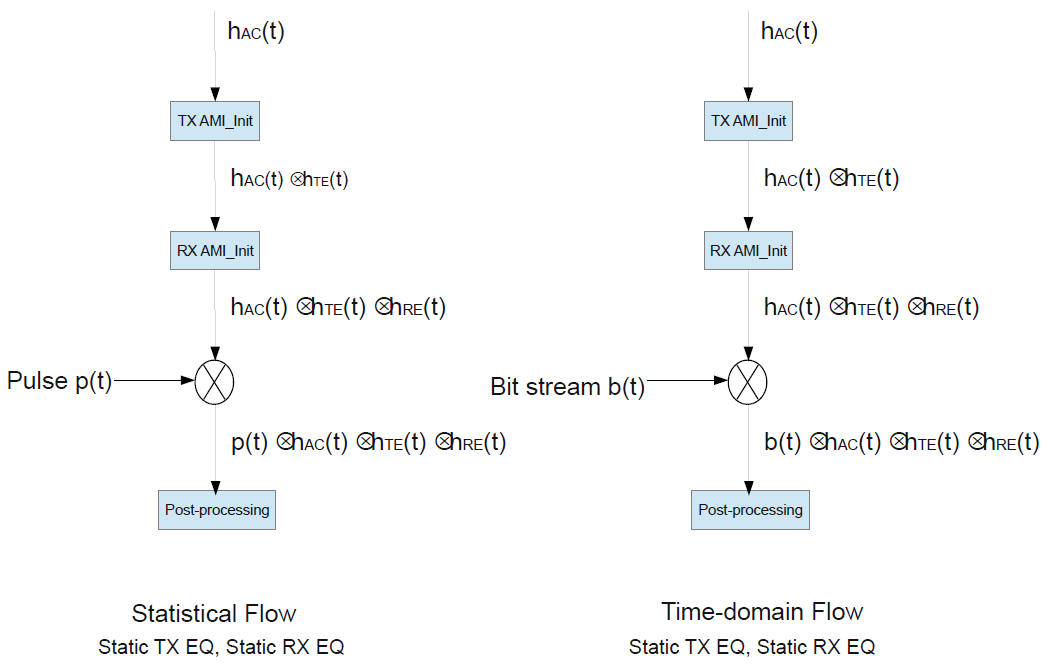#### Tx Init-Only and Rx GetWave-Only

The `AMI_Init` function of the RX model does not return an impulse response. So a statistical simulation only includes the TX model and the analog channel. This means that the final eye diagram is that of the signal at the output of the analog portion of the RX model which is often the die pad of the receiver device. In a time-domain simulation, an EDA tool must use the impulse response of the TX model so a time-domain eye includes the static TX equalization and the dynamic effect of the RX equalization.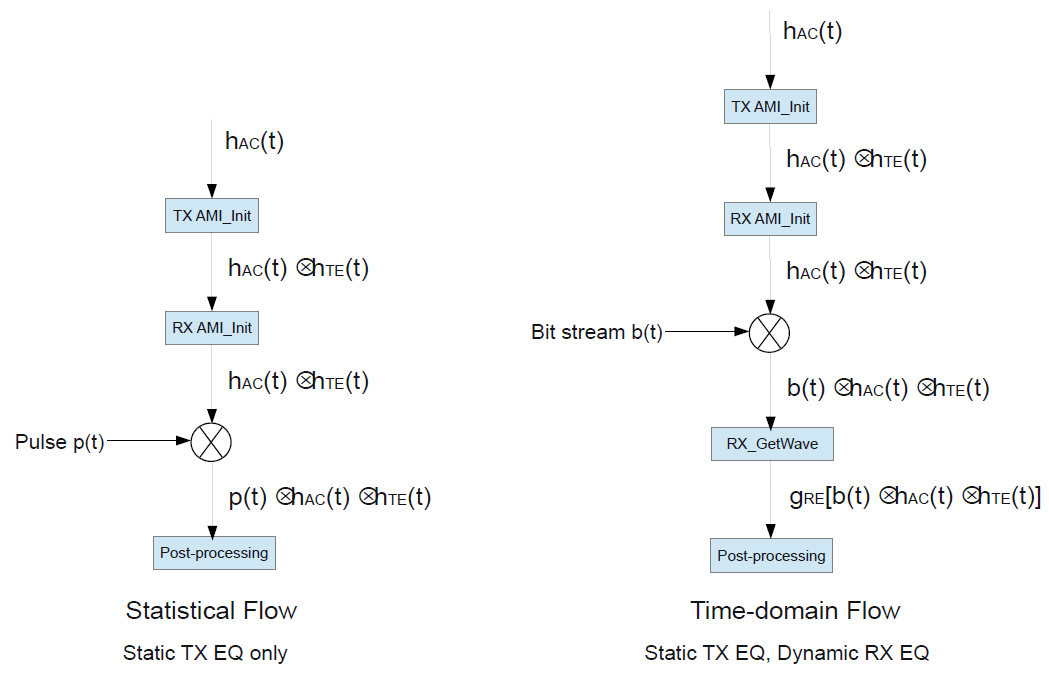#### Tx Init-Only and Rx Dual

You can perform a statistical simulation of the entire path. An EDA tool must use the impulse response of the TX model in a time-domain simulation.

The EDA tool is required to call the RX `AMI_Init` function, which returns an impulse response reflecting the RX equalization. The equalization is applied again by the `AMI_GetWave` function. So, the EDA tool must ensure that the RX equalization is not applied twice in a time-domain simulation.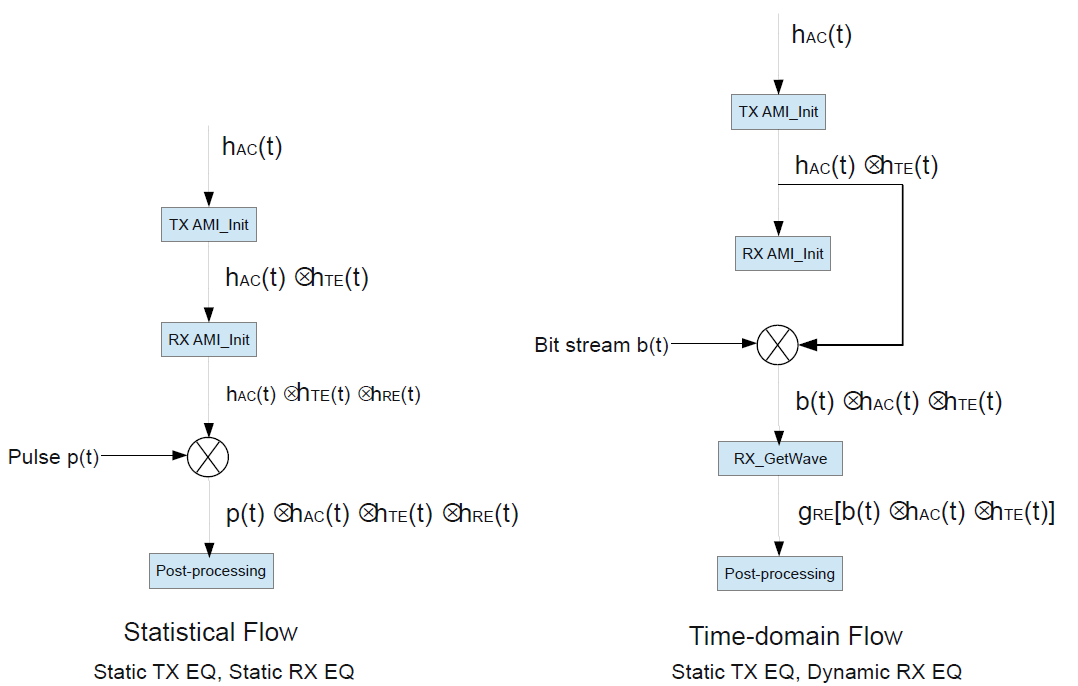This combination of models offers almost a full-featured statistical and time-domain simulations. The only limitation of the flow is the lack of the TX GetWave function and the ability to model dynamic TX equalization. However, TX equalization is generally static and you can choose between statistical and time-domain flows based on the goal of the simulation.

#### Tx GetWave-Only and Rx Init-Only

The `AMI_Init` function of the TX does not return an impulse response. As such, a statistical simulation shows only the effect of the analog channel and RX model. An EDA tool must use the impulse response of an RX model in a time-domain simulation.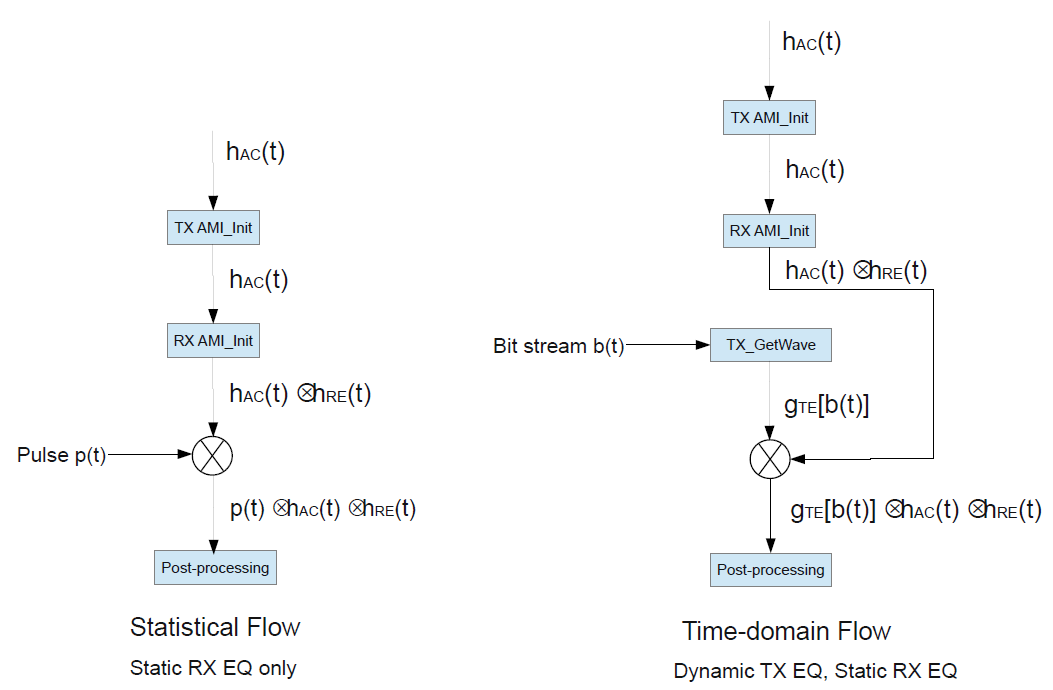#### Tx GetWave-Only and Rx GetWave-Only

Both TX and RX models provide `AMI_GetWave` functions, so you can perform full GetWave-based time-domain simulations. However, `Init_Returns_Impulse` is false for both models. As a result, a statistical simulation includes the analog channel characteristics only.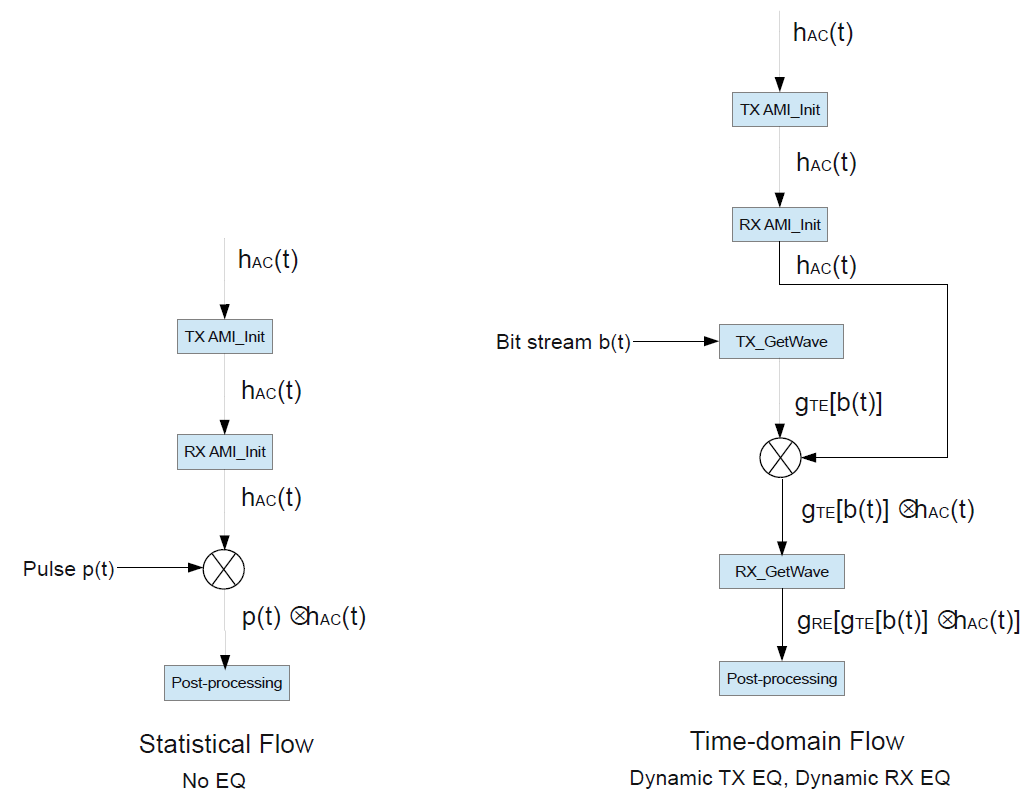#### Tx GetWave-Only and Rx Dual

Both TX and RX models provide `AMI_GetWave` functions, so you can perform full GetWave-based time-domain simulations. However, the EDA tool must ensure that the RX equalization is only applied by the `AMI_GetWave` function. `Init_Returns_Impulse` is false for the TX model. As a result, a statistical simulation includes only the analog channel and RX equalization.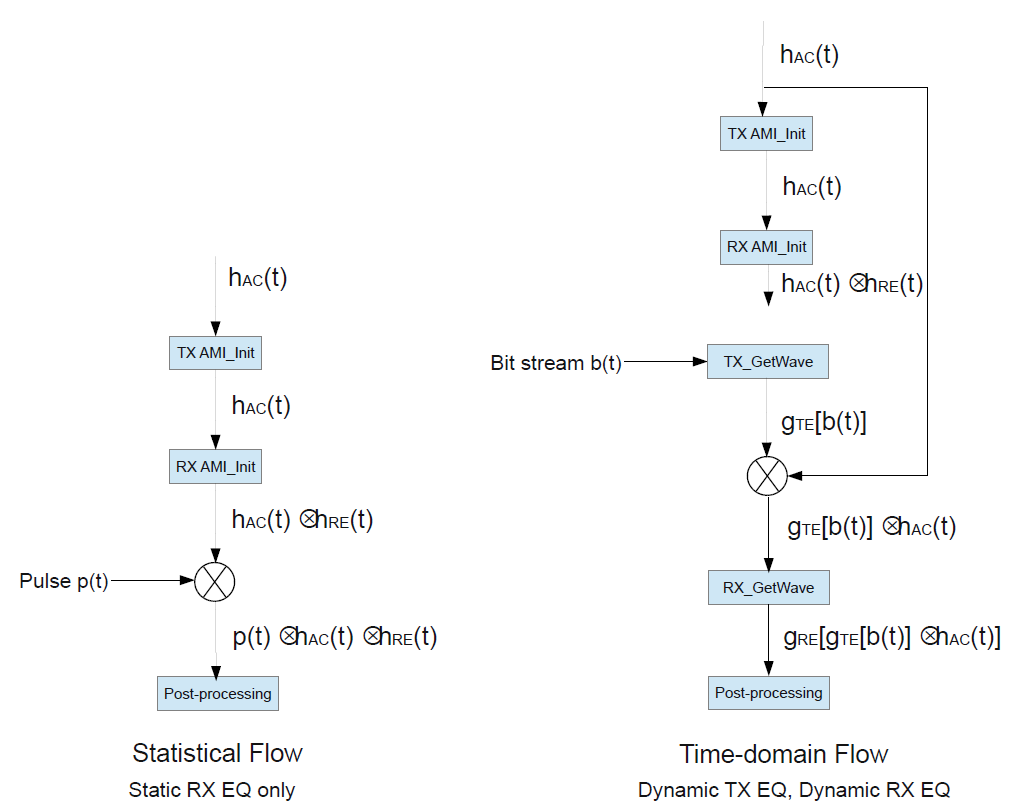#### Tx Dual and Rx Init-Only

`Init_Returns_Impulse` is true for both TX and RX models. So you can perform a full statistical simulation. The RX model does not include an `AMI_GetWave` function so the impulse response must be used in a time-domain simulation. The EDA tool must ensure that the TX equalization is only applied by the `AMI_GetWave` function.

This can present a problem in certain circumstances. For example, as the AMI specification notes, an RX `AMI_Init` function may include an optimization algorithm. In this case, the impulse response presented to the RX `AMI_Init` function must include the TX equalization. However, this leads to a double-counting of the TX equalization in a time-domain simulation.

A possible solution to this problem is an extra processing step involving deconvolution to obtain the impulse response of the RX equalization in isolation. This is then convolved with the impulse response of the active channel and the result is used in a standard GetWave-based simulation. If you are using an EDA tool, you should be aware that deconvolution tends to be numerically ill-behaved. You must exercise caution if the EDA tool implements this extra step.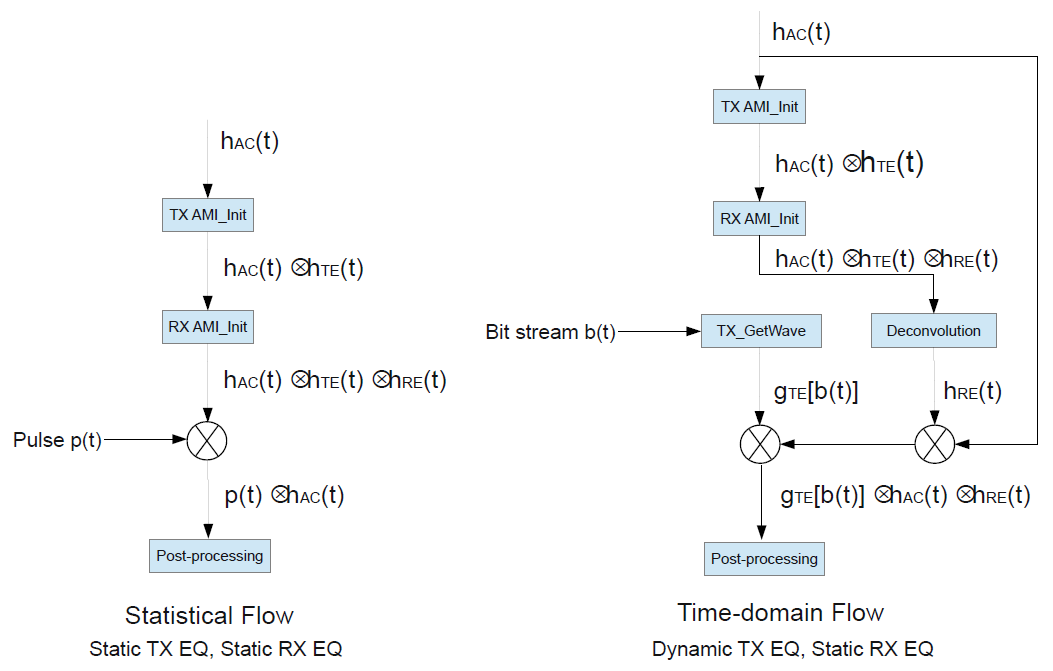#### Tx Dual and Rx GetWave-Only

`Init_Returns_Impulse` is false for the RX model. So a statistical simulation only shows the effects of the TX equalization and the active channel. This means that the final eye diagram is essentially that of the analog portion of the RX model with no RX equalization. `GetWave_Exists` is true for both models, so you can perform a full time-domain simulation. The EDA tool must ensure that the Init-based TX equalization is not applied in addition to that of the GetWave function.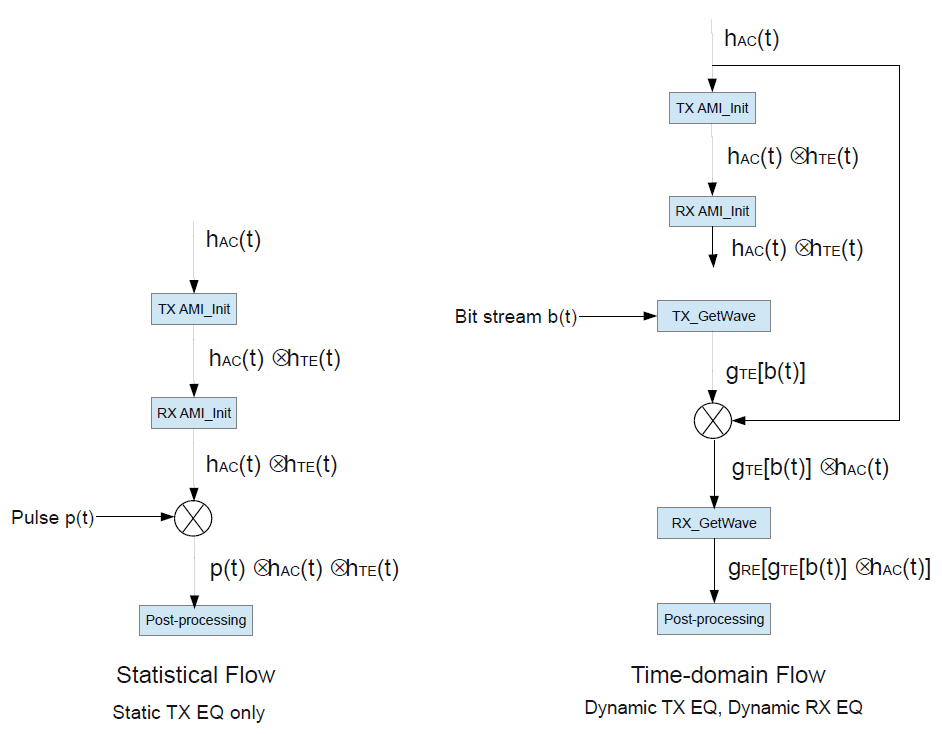#### Tx Dual and Rx Dual

Both TX and RX models are capable of statistical and time-domain simulations and you can select either with no limitations. This is the best possible case and the choice between statistical or time-domain flow depends on the goal of the simulation. The EDA tool must ensure there is no double-counting of equalization during a time-domain simulation.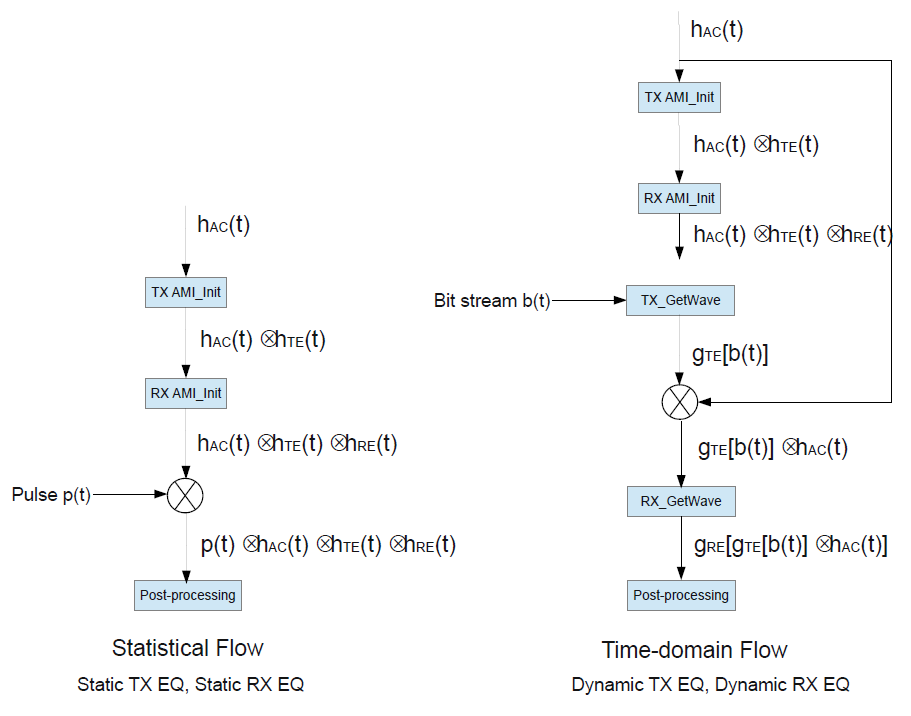#### Simulation Flow Summary

The main elements of the nine different flows can be consolidated into the single diagram: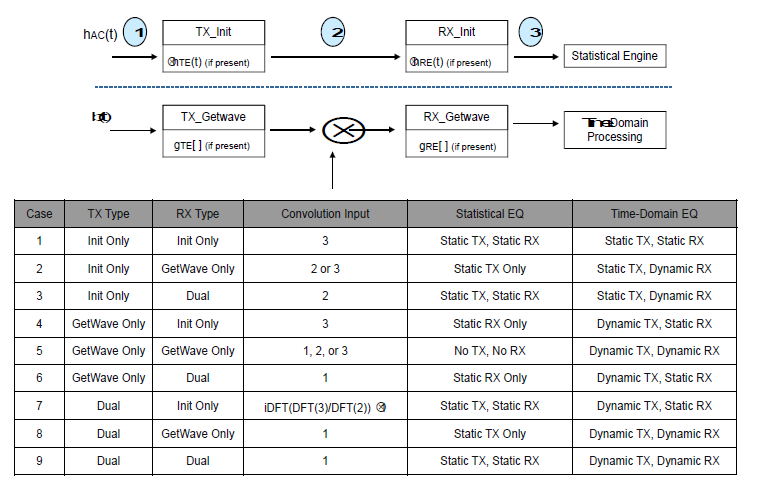The statistical flow consists of convolution of the impulse response of the analog channel with the impulse response of the TX equalization, if included in the model, and then convolution with the impulse response of the RX equalization, if included in the model.

The time-domain flow consists of application of the TX `AMI_GetWave` function, if it exists, followed by a convolution step, followed by application of the RX `AMI_GetWave` function, if it exists.

The impulse response used in the convolution step depends on the particular combination of TX and RX models. For example, in a time-domain simulation of Case 2, the impulse response corresponding to steps 2 or 3 of the statistical flow is used to represent the TX equalization.

Case 7 shows one implementation of the extra step required for deconvolution where the Discrete Fourier Transform (DFT) of the impulse response from step 3 of the statistical flow is divided by the DFT of the impulse response from step 2 to give the DFT of the RX equalization only. An inverse DFT transforms the frequency-domain representation of the equalization to an impulse response in the time-domain, to be convolved with the impulse response of the analog channel. This approach is based on the principle that deconvolution in the time domain is equivalent to division in the frequency domain.